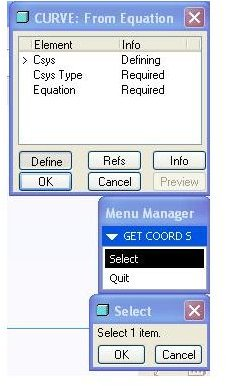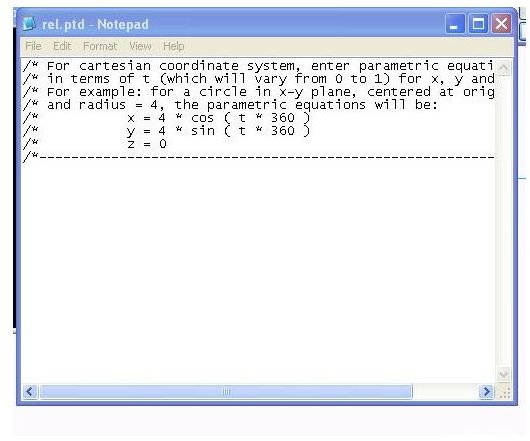# Creating a Pro-E Datum Curve Using Equation – Know the Method of Creating Pro-E Datum Curves with Equations

Pro E datum features are very useful for working with ProE surface modeling, Pro engineer sheet metal design, Pro-E solid modeling, and Pro Mechanica. There are many datum features creation methods in ProE. Like many other methods datum curves can be created using equations also. Below is the procedure for creating Pro-E datum curves with equations:

## Step-1

Run ProE. Go to insert>model datum>curve and select the option “equation” and you will get a window like below:## Step-2

Select the co-ordinate system about which you want to write the equation of the datum curve.

## Step-3

Next, you have to select the types of the co-ordinate system whether it is of Cartesian or cylindrical or spherical co-ordinate. And you will get the equation editor as below:## Step-4

Here you have to type the equation of the desired curves in parametric form. A parametric equation is an equation, equation of X, Y and Z is expressed in terms of a common parameter “t”. As you can see from the given example of creating the circular datum curve of radius 4 by typing the following codes below the dashed line of the equation editor:

x=4*cos(t*360)

y=4*sin(t*360)

z=0

## Step-5

Now, say you want to create an elliptical curve with major and minor axis equals to 10 and 6 respectively. In that case you have to write:

x=3*cos(t*360)

y=5*sin(t*360)

z=0

## Step-6

Now, say you need a datum curve like a helical spring. Let’s take the diameter of the helical spring as 10 and the height of the spring as 10. For creating such a Pro-E datum curve write the equations as below:

x=5*cos(t*360)

y=5*sin(t*360)

z=10

## Conclusion

Creating the Pro-E datum curves with equations requires knowledge of parametric equations of different geometric curves. The method of creating Pro-E datum curve using equation is useful for the cases, where you know the exact shape of the curve and you are in a position to explain the curve mathematically.

## Related Readings

Discussion about sketcher constraints in pro-engineer: You already know that, this 3D CAD modeling software especially pro engineer is parametric in natures. Sketcher constraints are one of the reasons why 3D CAD modeling software are parametric. How? Let’s discuss in details about sketcher constraints of pro engineer in this article.

Working with Parameters and Relations in Pro Engineer: If you take the example of an IC engine, the parameters are like piston, crank shaft and relation is like the connecting rod. Pro engineer parameters and relations help to keep the design intent intact. In this article we will see how to create and use parameters and relations in pro engineer.